# 概率论的一些基本问题

hit2015spring $\frac{hit2015spring}{晨凫追风}$

## 条件概率

A $A$B$B$是任意两个事件，且 P(B)>0 $P(B)>0$，则称 P(AB)P(B) $\frac {P(AB)}{P(B)}$为事件 A $A$在事件B$B$发生的条件下发生的条件概率。记作：

P(A|B)=P(AB)P(B)

（两个事件的积的概率）=（其中一个事件的概率） × $\times$(另一个事件在前面一个事件发生条件下的条件概率)即：

P(AB)=P(A|B)P(B)=P(B|A)P(A)

P(A1A2An)=P(A1)P(A2|A1)P(A3|A1A2)P(A4|A1A2A3)P(An|A1A2A3,An)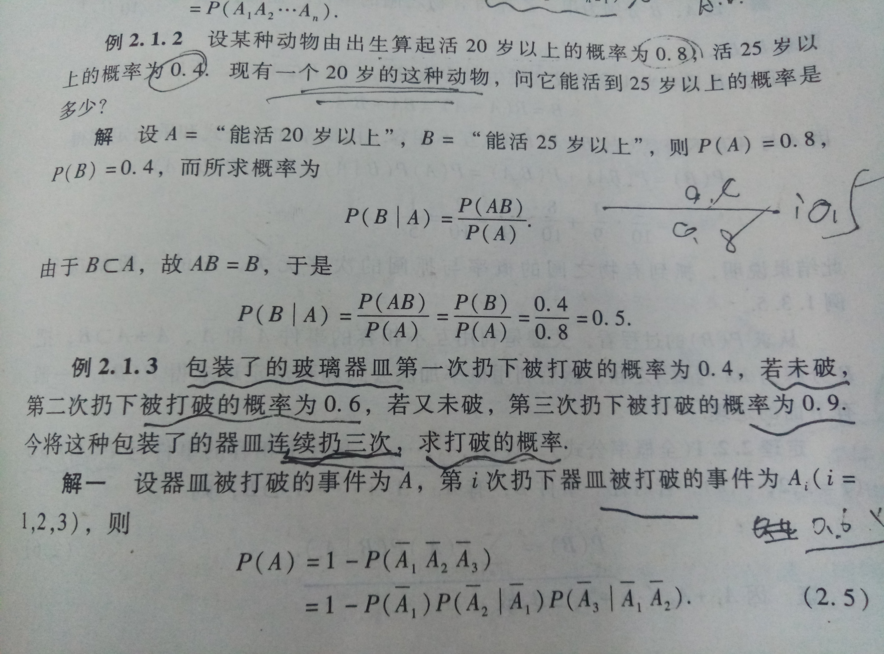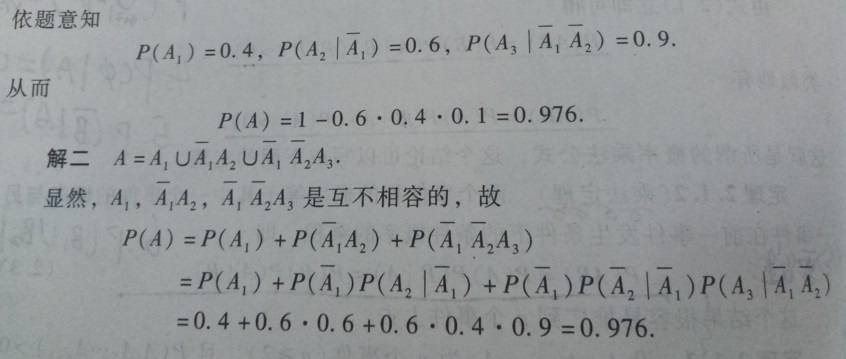### 全概率公式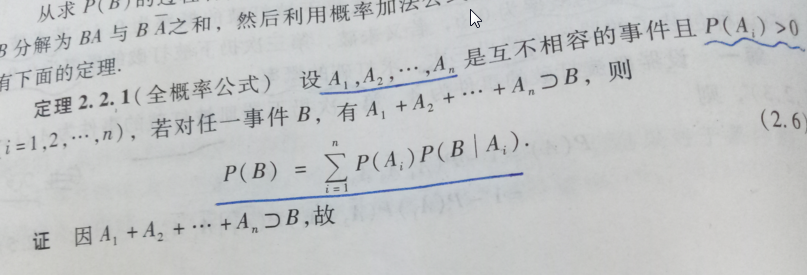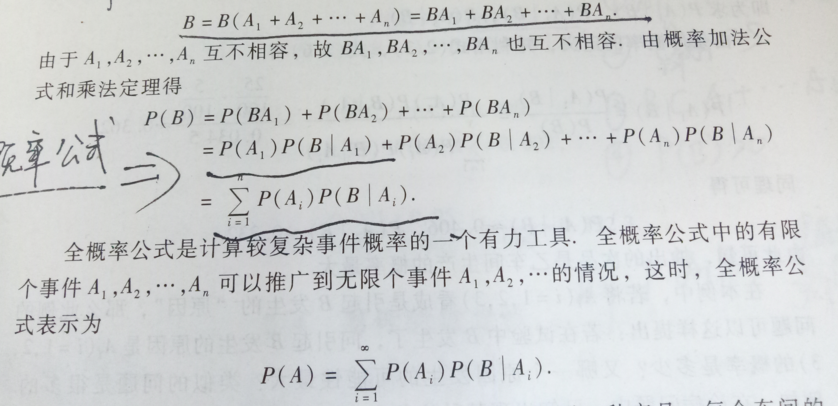### 贝叶斯公式

P(Ai|B)=P(AiB)P(B)=P(B|Ai)P(Ai)P(B)=P(B|Ai)P(Ai)i=1nP(B|Ai)P(Ai)

P(B|Ai)=P(AiB)P(Ai)

P(Ai) $P(A_i)$是先验概率，一般是经验的总结。 P(Ai|B) $P(A_i|B)$是后验概率，表示实验之后各种原因发生的可能性

### 重复独立实验、二项概率公式

Pn(k)=Cknpk(1p)nk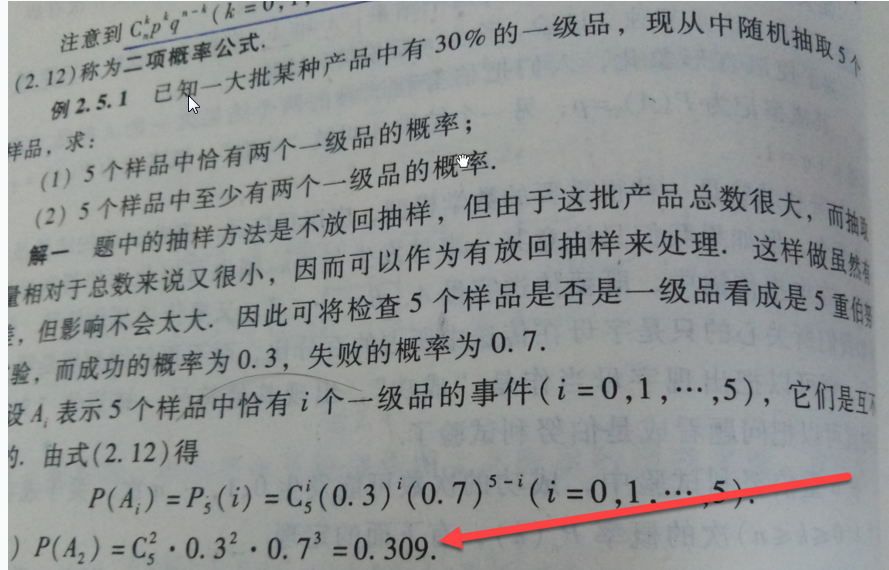Cknpk(1p)nkλkk!eλ

### 数学期望和方差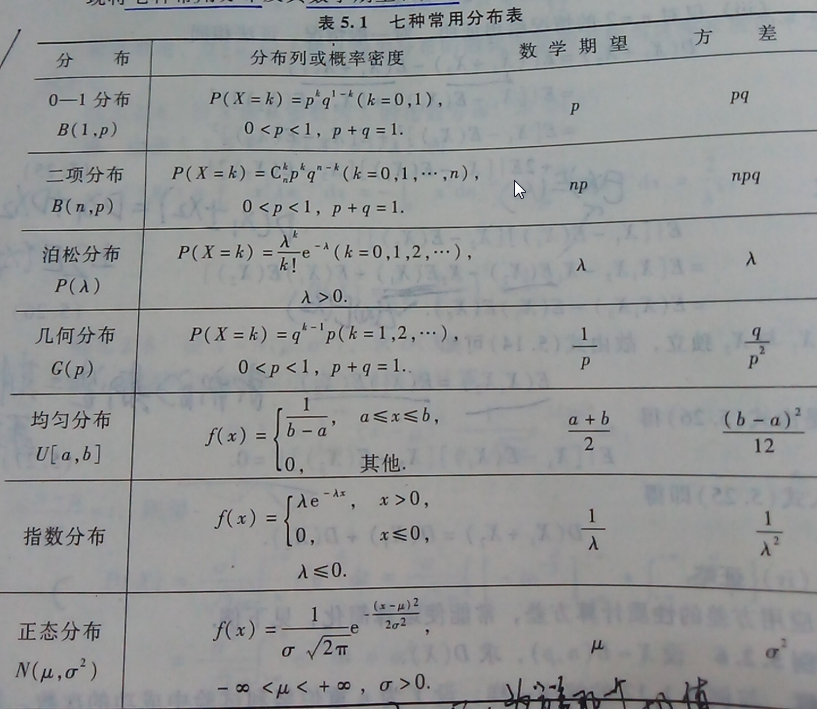0-1分布泊松分布几何分布

### 切比雪夫不等式

P[|XE(X)|ε]D(X)ε2

### 伯努利大数定律

n $n$重伯努利实验中，成功的次数为Yn$Y_n$,而每次成功的概率为 p(0<p<1) $p(0< p < 1)$,则对任意的 ε>0 $\varepsilon>0$

limnP(|Ynnp|ε)=0

### 辛钦大数定律

limnP(|1ni=1nXiμ|ε)=0

1ni=1nXi $\frac{ 1 }{ n }\sum\limits_{ i=1 }^{ n } X_i$ 是随机变量 X $X$n$n$个观测值的算术平均值，而 μ=E(X) $\mu=E(X)$,当实验次数足够大的时候平均值与数学期望之差的绝对值不小于任一指定的正数 ε $\varepsilon$的概率小于任意一个预先指定的正数，这就是算术平均值稳定性的解释

### 中心极限定理

Lindeberg-levi 中心极限定理

limnP(1nσ(i=1mXinμ)0)=x12πet22dt

n $n$重伯努利实验中，成功的次数为Yn$Y_n$而在每次实验中成功的概率是 p $p$则对一切的x$x$

limnP(Ynnpnpqx)=x12πet22dt=Φ(x)

03-041万+01-127265
03-056094
01-194万+
10-28851
08-25160
06-11258
05-18747
08-101764
10-232万+